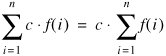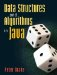# C.6. Constant Factors

A constant factor can be moved to the outside of a sum.This is illustrated in Figure C-5.

Figure C-5. On the left, we multiply each term by a constant (3) before adding. On the right, we evaluate the sum and then multiply by the constant. The area is the same.Data Structures and Algorithms in Java
ISBN: 0131469142
EAN: 2147483647
Year: 2004
Pages: 216
Authors: Peter Drake